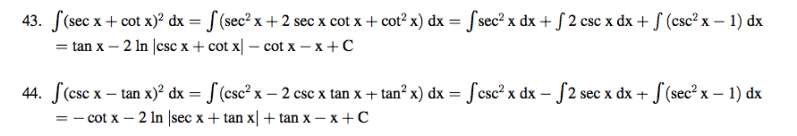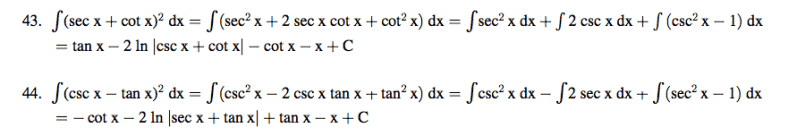# Trigonometric antiderivatives

## Homework StatementI don't see how they're going from

sec x cot x to csc x

and

csc x tan x to sec x

The derivative of sec x is sec x tan x not csc x tan x
and the derivative of csc x is -csc x cot x

and if it's an identity then they didn't care to inform me of that at this website

http://www.sosmath.com/trig/Trig5/trig5/trig5.html

Curious3141
Homework Helper

## Homework StatementI don't see how they're going from

sec x cot x to csc x

and

csc x tan x to sec x

Express sec x, csc x, cot x and tan x in terms of sin x and cos x first.

The derivative of sec x is sec x tan x not csc x tan x
and the derivative of csc x is -csc x cot x

Sure, but what's the relevance of this? They weren't integrating at that stage, merely simplifying using trig identities.

eumyang
Homework Helper

## Homework StatementI don't see how they're going from

sec x cot x to csc x

and

csc x tan x to sec x

The derivative of sec x is sec x tan x not csc x tan x
and the derivative of csc x is -csc x cot x

and if it's an identity then they didn't care to inform me of that at this website

http://www.sosmath.com/trig/Trig5/trig5/trig5.html
You should not only recognize the trig identities, but different variations of them. For instance,
$\tan \theta = \frac{\sin \theta}{\cos \theta}$.
But it is also true that
$\tan \theta = \sin \theta \sec \theta$.